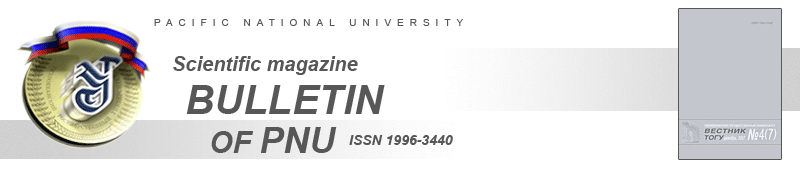UDC 539.186

# The description of (e,3e) reaction with a helium atom on the basis of the solution of the Phadeev-Merkuriev equation in J-matrix approach

The version of the J-matrix method is developed to solve the three-body differential Faddeev-Merkuriev equations. To check the efficiency of our method the calculations of fivefold differential cross section for electron-impact double ionization of helium are performed. The results are in good agreement with the experiment both in shape and in absolute values.

References:

1. Heller E. J. and Yamani H. A. New L2 approach to quantum scattering: Theory // Phys. Rev. A. 1974. V. 9; Yamani H. A. and Fishman L. J-matrix method: Extensions to arbitrary angular momentum and to Coulomb scattering // J. Math. Phys. 1975. V. 16; Broad J. T. and Reinhardt W. P. One- and two-electron photoejection from H-: A multichannel J-matrix calculation // Phys. Rev. A. 1976. V. 14.
2. Filippov G. F., Okhrimenko I. P. O vozmozhnosti ispolzovaniya ostsillyatornogo bazisa dlya resheniya zadach nepreryvnogo spektra // YAF. 1980. T. 32.
3. Smirnov Yu. F., Nechaev Yu. I. The elements of scattering theory in the harmonic oscillator representation // Kinam. 1982. V. 4; Smirnov YU. F., Nechaev YU. I. O reshenii zadachi rasseyaniya v ostsillyatornom predstav-lenii // YAF. 1982. T. 35.
4. Revai J., Sotona M. and Zofka J. Note on the use of harmonic-oscillator wavefunctions in scattering calculations // J. Phys. G. 1985. V. 11.
5. Knyr V. A., Stotland L. YA. Problema trekh tel i metod J-matritsy // YAF. 1992. T. 55; Knyr V. A., Stotland L. YA. O vozmozhnosti resheniya zadachi trekh tel metodom J-matritsy // YAF. 1996. T. 59.
6. Bray I. and Steblovics A. T. Convergent close-coupling calculations of electron-hydrogen scattering // Phys. Rev. A. 1992. V. 46.
7. Zaytsev S. A., Knyr V. A., Popov YU. V. Reshenie uravneniya Fad-deeva-Merkureva v J-matrichnom podkhode: primenenie k kulonovskim zadacham // YAF. 2006. T. 69. № 2. (to be published).
8. Papp Z., Hu C.-Y., Hlousek Z. T., Konya B. and Yakovlev S. L. Three-potential formalism for the three-body scattering problem with attractive Coulomb interactions // Phys. Rev. A. 2001. V. 63.
9. Merkurev S. P., Faddeev L. D. Kvantovaya teoriya rasseyaniya dlya sistem neskolkikh chastits. M., 1985.
10. Kheifets A., Bray I., Lahmam-Bennani A., Duguet A. and Taouil I. A comparative experimental and theoretical investigation of the electron-impact double ionization of He in the keV regime // J. Phys. B. 1999. V. 32.
11. Knyr V. A., Nasyrov V. V., Popov YU. V. Metod J-matritsy v primenenii k opisaniyu  -reaktsii na atome geliya // ZHETF. 2001. T. 119. Vyp. 5.
12. Kvitsinsky A. A., Wu A., Hu Chi-Yu. Scattering of electrons and positrons on hydrogen using the Faddeev equations // J. Phys. 1995. V. 28.
13. Heller E. J. Theory of J-matrix Greens functions with applications to atomic polarizability and phase-shift error bounds // Phys. Rev. A. 1975. V. 12.Сontents